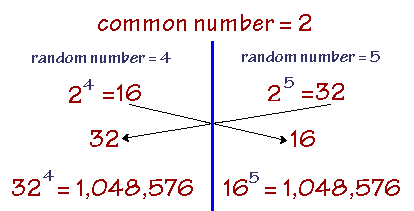# Diffie-Hellman

Also found in: Acronyms.

## Diffie-Hellman

(cryptography)
A public-key encryption key exchange algorithm.

FAQ.

## Diffie-Hellman

A cryptographic key exchange method developed by Whitfield Diffie and Martin Hellman in 1976. Also known as the "Diffie-Hellman-Merkle" method and "exponential key agreement," it enables parties at both ends to derive a shared, secret key without ever sending it to each other.

Using a common number, both sides use a different random number as a power to raise the common number. The results are then sent to each other. The receiving party raises the received number to the same random power they used before, and the results are the same on both sides. See elliptic curve cryptography and key management.

Very CleverThere is more computation in actual practice, but this example, which uses tiny numbers to illustrate the concept, shows a very clever mathematical approach. Each party raises the common number, which is 2 in this example (this has nothing to do with binary-- it is just the number "2") to a random power and sends the result to the other. The received number is raised to the same random power. Note that both parties come up with the same secret key, which was never transmitted intact.
Copyright © 1981-2019 by The Computer Language Company Inc. All Rights reserved. THIS DEFINITION IS FOR PERSONAL USE ONLY. All other reproduction is strictly prohibited without permission from the publisher.
References in periodicals archive ?
(Computational Diffie-Hellman (CDH) Problem) over an elliptic curve.
In additional, to prove the security of dCRKS system, we need to introduce a new hard problem on G and [G.sub.T], namely, the Strong Decisional Diffie-Hellman assumption (in short SDDH).
Elliptic curve Diffie-Hellman is an is an obscure key conformity protocol that permits two clients, each having an elliptic bend public-private key combine off, to create a mutual undisclosed over an uncertain channel.
Bernstein, "Curve25519: New Diffie-Hellman Speed Records", in Public Key Cryptography (PKC 2006), Lecture Notes in Computer Science, vol.
An adversary who intercepts R, z, s, T, g, h, and C between the reader and the tag and attempts to obtain the tag ID cannot get the session key KTR, because this is computationally infeasible under the Diffie-Hellman problem and the elliptic curve discrete logarithm problem (ECDLP).
Definition 2 (computational Diffie-Hellman (CDH) assumption).
For commonly used 1024-bit keys, it would take about a year and cost a "few hundred million dollars" to crack just one of the extremely large prime numbers that form the starting point of a Diffie-Hellman negotiation.
Elliptic Curve Computational Diffie-Hellman Problem (ECCDHP): Given G and two point xG, yG, computation of xyG is hard, where x, y [member of] [Z.sub.p.sup.*] and are randomly chosen and are smaller than n.
Assuming the hardness of computational Diffie-Hellman problem over groups in bilinear maps, the proposed CLAS scheme is proven secure in random oracle model, Bellare and Rogaway (1993).
(2.) Rouse, M., "Diffie-Hellman key exchange (exponential key exchange)," TechTarget, August 2007.

Site: Follow: Share:
Open / Close